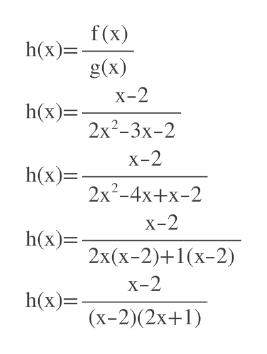# For f(x) = x - 2 and g (x) = 2x2 - 3x - 2, state the domain of  h(x) = f(x)/g(x). Write your answer using interval notation.

Question
3 views

For f(x) = x - 2 and g (x) = 2x2 - 3x - 2, state the domain of  h(x) = f(x)/g(x). Write your answer using interval notation.

check_circle

Step 1

h(x)=f(x)/g(x)

Denominator can not be zero.

S...help_outlineImage Transcriptionclosef(x) h(x)= g(x) х-2 h(x)= 2x?-3х-2 X-2 h(x)= 2x?-4х+x-2 x-2 h(x)= 2x(х-2)+1(х-2) х-2 h(x)= (х-2)(2х+1) fullscreen

### Want to see the full answer?

See Solution

#### Want to see this answer and more?

Solutions are written by subject experts who are available 24/7. Questions are typically answered within 1 hour.*

See Solution
*Response times may vary by subject and question.
Tagged in

### Other